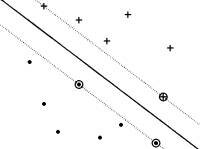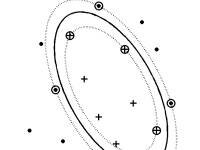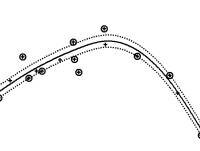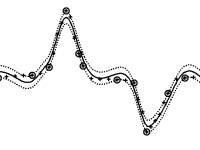HomeSupport Vector Machines SVM ReviewsSVM BooksSVM SoftwarePattern RecognitionOptimum HyperplaneSVM Regressionν-SVMSVM StatisticsMachine Learning MLnetNEuroNetEvoNetUCI RepositoryList 1List 2List 3WikipediaRepositoryROCKITWekaC4.5YALETutorialsSVM - Support Vector Machines
 Optimum Separation Hyperplane

The optimum separation hyperplane (OSH) is the linear classifier with the maximum margin for a given finite set of learning patterns. The OSH computation with a linear support vector machine is presented in this section.Figure 1. The optimum separation hyperplane (OSH).

Consider the classification of two classes of patterns that are linearly separable, i.e., a linear classifier can perfectly separate them (Figure 1). The linear classifier is the hyperplane H (wx+b=0) with the maximum width (distance between hyperplanes H1 and H2). Consider a linear classifier characterized by the set of pairs (w, b) that satisfies the following inequalities for any pattern xi in the training set:These equations can be expressed in compact form asorBecause we have considered the case of linearly separable classes, each such hyperplane (w, b) is a classifier that correctly separates all patterns from the training set:For all points from the hyperplane H (wx + b = 0), the distance between origin and the hyperplane H is |b|/||w||. We consider the patterns from the class -1 that satisfy the equality wx + b = -1, and determine the hyperplane H1; the distance between origin and the hyperplane H1 is equal to |-1-b|/||w||. Similarly, the patterns from the class +1 satisfy the equality wx + b = +1, and determine the hyperplane H2; the distance between origin and the hyperplane H2 is equal to |+1-b|/||w||. Of course, hyperplanes H, H1, and H2 are parallel and no training patterns are located between hyperplanes H1 and H2. Based on the above considerations, the distance between hyperplanes (margin) H1 and H2 is 2/||w||.

From these considerations it follows that the identification of the optimum separation hyperplane is performed by maximizing 2/||w||, which is equivalent to minimizing ||w||2/2. The problem of finding the optimum separation hyperplane is represented by the identification of (w, b) which satisfies:for which ||w|| is minimum.

 Search "SVM" in: PubMedPubMed CentralCiteSeerGoogle ScholarGoogleScirusBioChem PressSearch "Support Vector" in: PubMedPubMed CentralCiteSeerGoogle ScholarGoogleScirusBioChem PressJournals JMLRIEJMDBioinformaticsNucleic Acids ResearchBioMed CentralLiterature Databases PubMedPubMed CentralCiteSeerSearch Engines DOAJScirusOJOSE